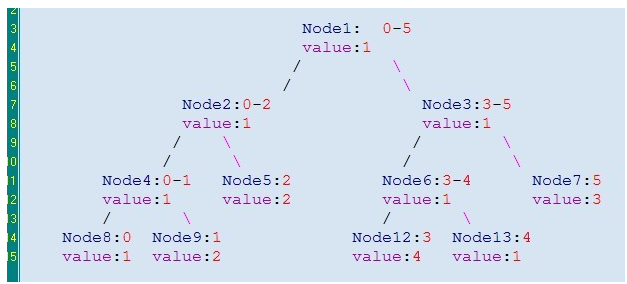#### wu等　　级 排　　名 2 经　　验 28964 参赛次数 8 文章发表 265 年　　龄 23 在职情况 学生 学　　校 电子科技大学 专　　业 通信工程

自我简介：

#### 你可能喜欢

 C语言程序设计教程（第三版）课后习题8.2 （C语言代码）浏览：867 数字整除 （C语言代码）浏览：627 （格式错误） qq290048663 碰巧告知我吧浏览：488 C二级辅导-进制转换 （C语言代码）浏览：628 C语言程序设计教程（第三版）课后习题9.8 （C语言代码）浏览：471

这个题解是我从博客上摘抄下来的

(a+b)/2即为mid,如此这样一直分支下去 直到每个结点中包含的线段成了一个点  线段树便构建好了#include <iostream>
#include <string.h>
#include <algorithm>
#include <cstdio>
#include <math.h>
using namespace std;
const int N=100000+10;
struct Node{
int l,r,v;
}node[N*4]; //线段树的每个节点  其中包括三个参数 l为线段左端点的值 r:线段右端点的值  v：该节点所存储的值
void Init(int now,int le,int ri){    //构建线段树 并将结点的值初始化全部为0
if(le==ri){
node[now].l=le;
node[now].r=ri;
node[now].v=0;
return;
}//如果线段的左端点==线段的右端点  那么即是最后的叶子结点 return
int mid=(le+ri)>>1;  //求出中点
int ne=now<<1;    //将结点的序号*2；
Init(ne,le,mid);  //构建左子树
Init(ne+1,mid+1,ri);  //构建右子树
node[now].l=le;  //给当前结点的线段左右端点赋值
node[now].r=ri;
node[now].v=0;  //给结点赋值
}
void insert(int now,int le,int ri,int add){       //在线段树中寻找对应的区间  给对应该区间的结点加上该发的

if(node[now].l==le&&node[now].r==ri){
return;
}  //给对应该区间的结点加上发的苹果数
int mid=(node[now].l+node[now].r)>>1;//求出中点
int ne=now<<1;  //结点的序号*2，方便构建新的结点
if(ri<=mid){
}
else if(le>mid){
}
else
{
}
}
void print(int now){
if(node[now].l==node[now].r){
cout<<node[now].v<<" ";
return;
}   //如果是最后的叶子结点 输出小朋友的苹果总数
int ne=now<<1;
//父亲的范围大 包含的儿子结点的区间   所以父亲下面儿子结点也要加上父亲结点被发的苹果数
node[ne].v+=node[now].v;
print(ne);
node[ne+1].v+=node[now].v;
print(ne+1);
}
int main(){
int n,m,i,a,b,c;
while(cin>>n>>m){
Init(1,1,n);
for(i=0;i<m;i++){
cin>>a>>b>>c;
insert(1,a,b,c);
}
print(1);
}
return 0;
}

0.0分

2 人评分

#### 评论区

棒棒哒！！
2018-03-11 21:31:20 | |
• «
• 1
• »

#### 精彩推荐

 简单的a+b （C语言代码）浏览：459 川哥的吩咐 （C++代码）浏览：602 A+B for Input-Output Practice (IV) （C++代码）浏览：508 十->二进制转换 （C语言代码）浏览：1100 C语言程序设计教程（第三版）课后习题4.9 （C语言代码）浏览：429 C语言程序设计教程（第三版）课后习题10.7 （C语言代码）浏览：380 C语言程序设计教程（第三版）课后习题6.6 （C++代码）浏览：464 DNA （C语言代码）浏览：417 A+B for Input-Output Practice (IV) （C语言代码）浏览：404 蚂蚁感冒 （C语言代码）浏览：554